Adding and subtracting integers can be a challenge for many students. When students struggle with this idea, we often resort to memory tricks. Unfortunately, when students only know how to use tricks and lack the necessary conceptual understanding to apply concepts to new ideas, students struggle.

Math is visual. Let’s teach it that way!

In the following videos and visual prompt images, students are implicitly introduced to the idea of the zero principle – the understanding that when you add an equivalent quantity of positive and negative values together, the result is always zero.

While these visual prompts may seem simplistic at first, gaining a firm grasp in this area is necessary in order for students to conceptualize more difficult integer operation ideas like subtracting negative values.

## Math Is Visual Prompts

Feel free to use the video and pause in-between ideas or alternatively, use the following visual prompts.

#### Visual #1 Prompts

Show the following image: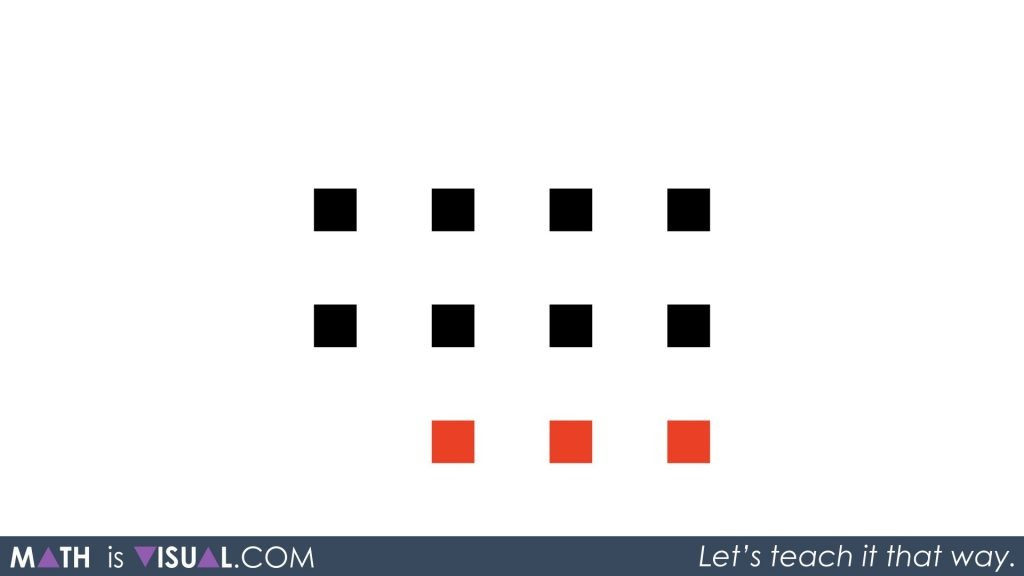You may consider working only with the visual prior to introducing the numerical symbols included in the visual below: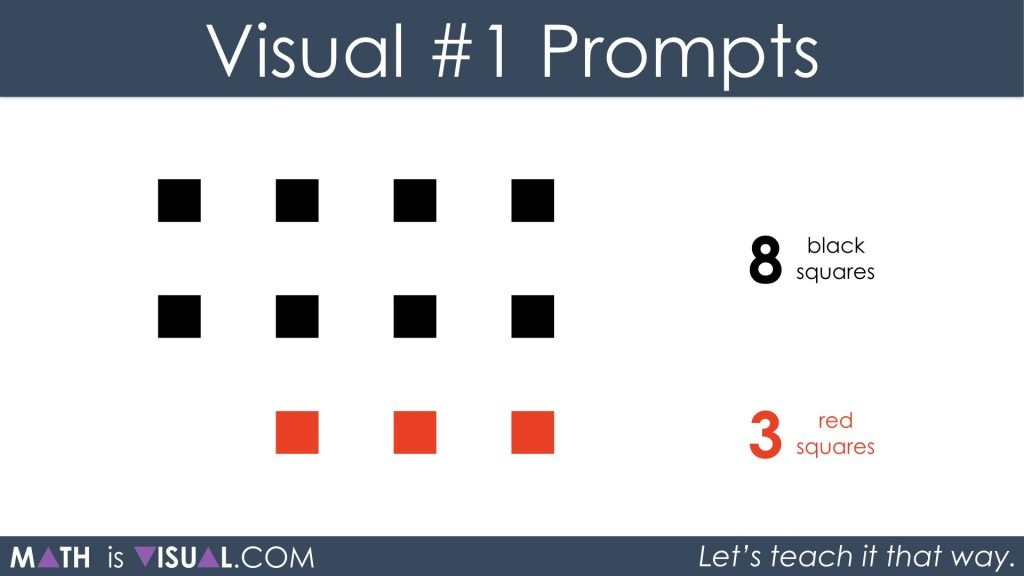After giving students some time to determine how many squares will remain and what colour they will be, you can show them the visual that matches this scenario.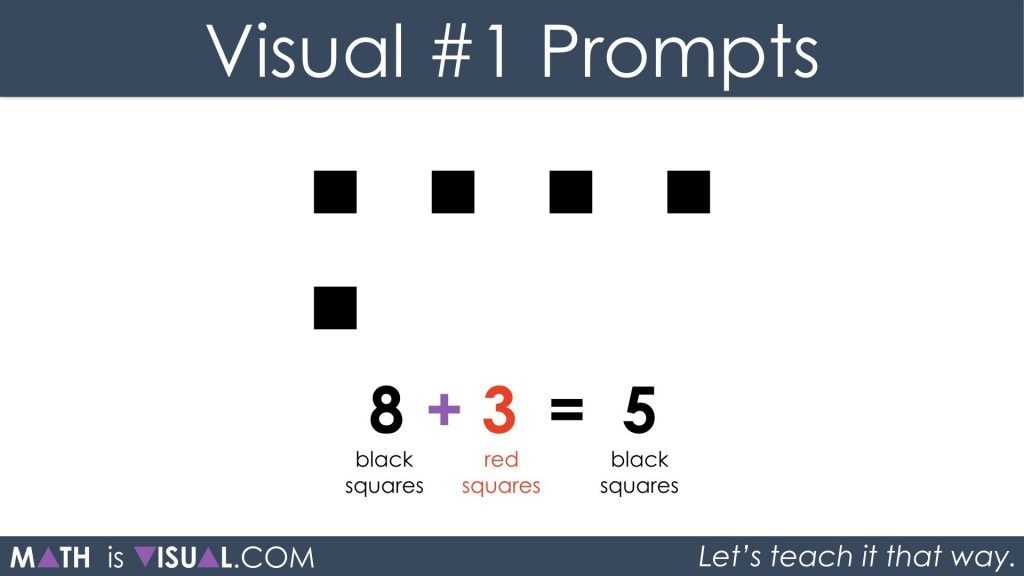#### Visual #2 Prompts

Show the following image: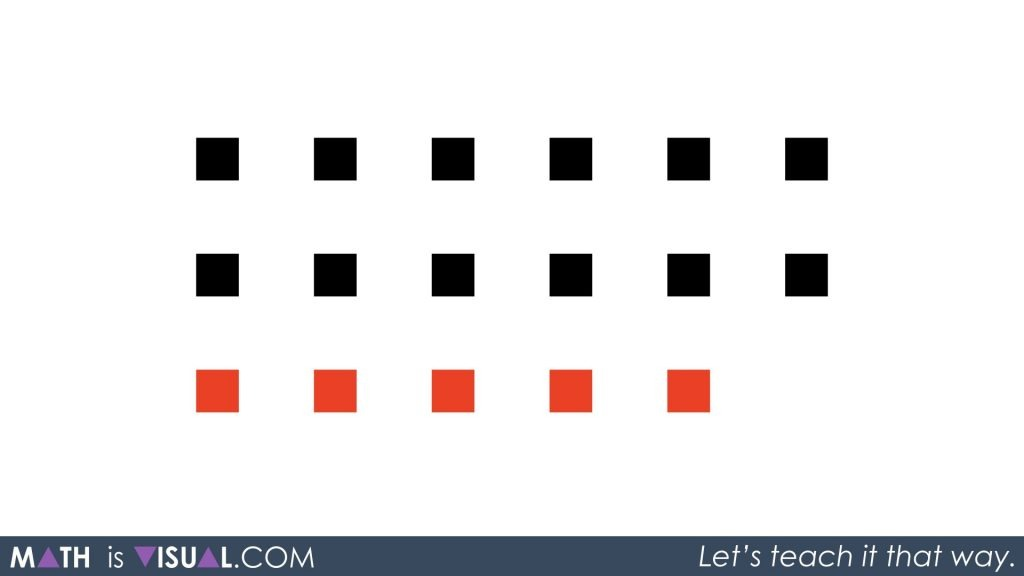After students use square tiles and come to their conclusion, have them write out their thinking using a numerical expression.

Then, show them this: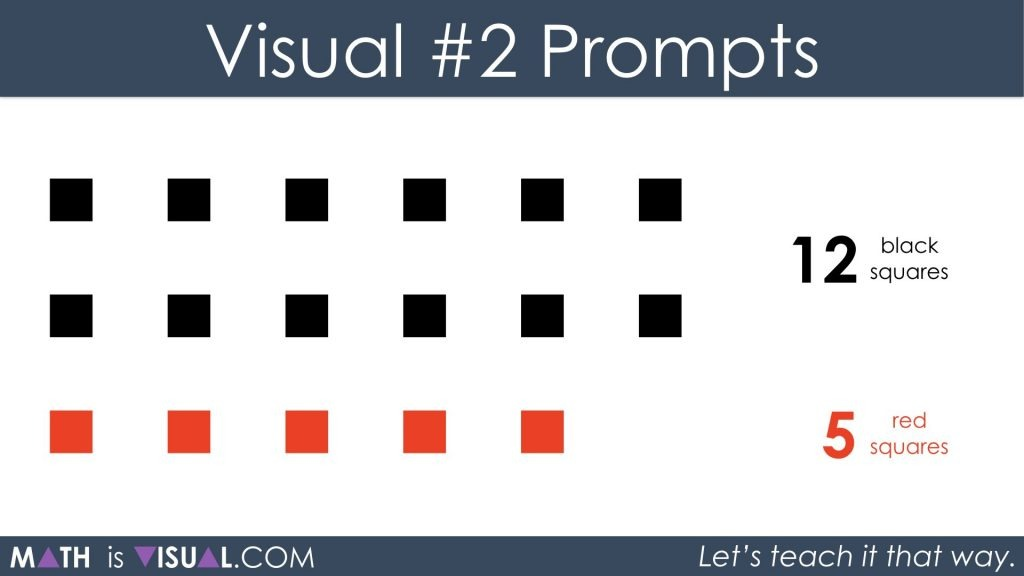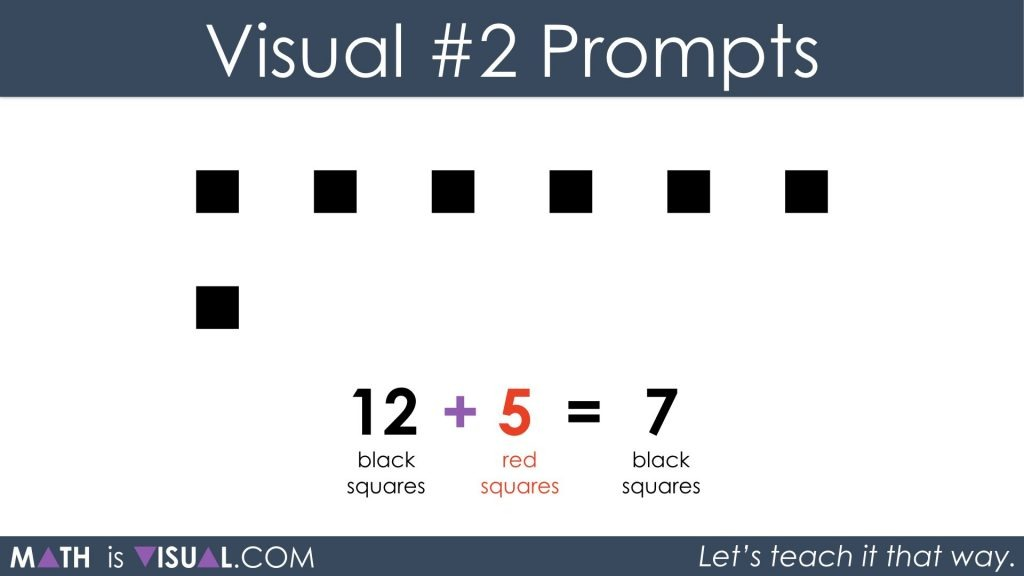#### Visual #3 Prompts

Show the following image: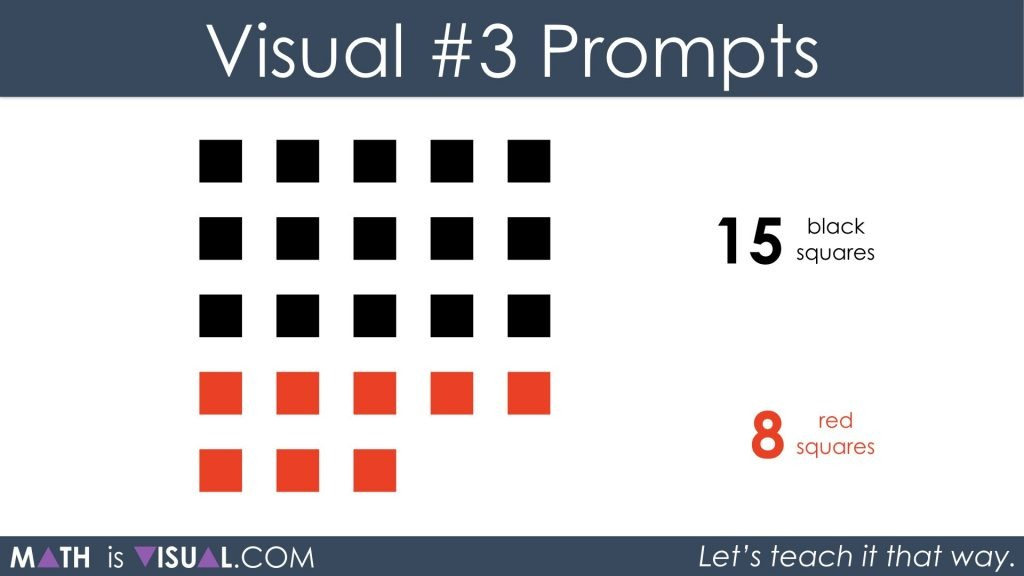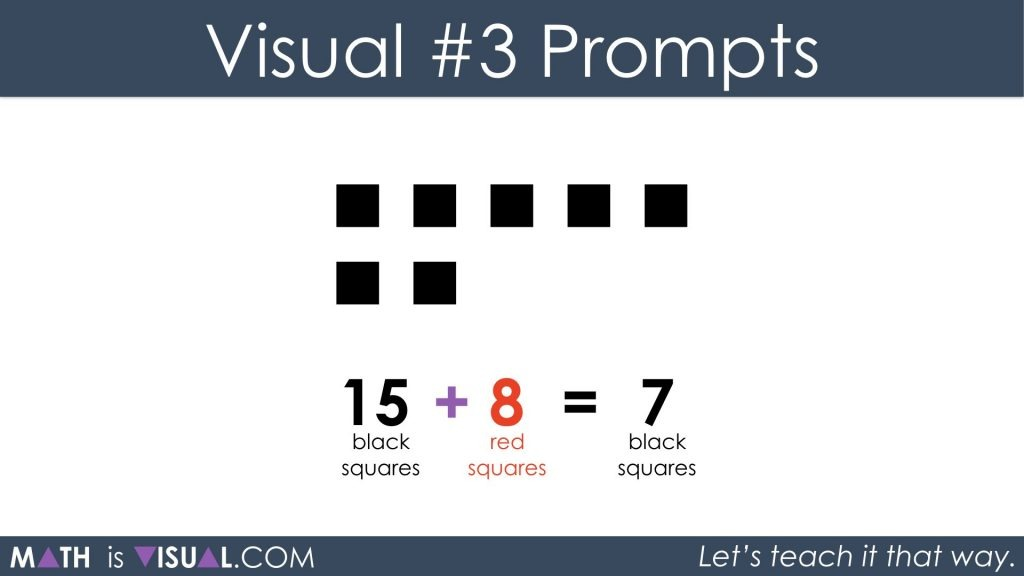#### 1 comment

•Patrick says:

Thank you for the help